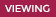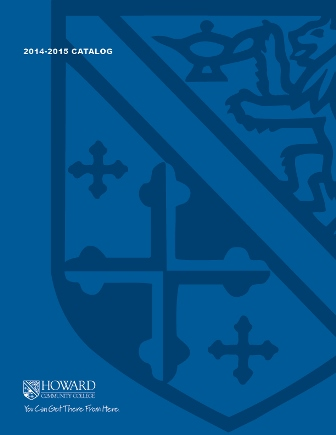2019-2020 College Catalog# MATH 240 Calculus III

Students will develop skills necessary to conclude the calculus sequence. The course includes vector calculus in both two- and three-dimensional space along with the classical theorems of Green, Stokes, and Gauss. It will also include partial derivatives and multiple integrals along with a number of appropriate applications. A graphing calculator and MATLAB, a computer algebra system, will be integral parts of the course.

4

### Prerequisite

MATH 182 or equivalent with a grade of C or higher

4 hours weekly

### Course Objectives

1. Evaluate elementary vector arithmetic expressions.
2. Evaluate and manipulate the dot and cross products of vectors
3. Formulate parametric equation of lines.
4. Formulate the point normal equation of a plane
5. Completely describe the features of any quadratic surface.
6. Solve classical engineering and physics problems using vector calculus.
7. Understand and use the chain rule, for functions of two/three variables, to solve problems
8. Apply the concepts of multiple integration to solve complex volume, area, density,
centroid problems.
9. Understand and apply the gradient concept to solve problems relative to conservative force fields.
10. Solve simple problems using the concepts of Green/Strokes Theorem and the Divergence
Theorem.
11. Use the computer algebra system, MATLAB, to communicate, collect, display, and analyze information and efficiently solve problems.

### Course Objectives

Evaluate elementary vector arithmetic expressions.

#### Learning Activity Artifact

• Other (please fill out box below)
• Final exam

#### Program Goal(s)

Degree: Actuarial Science - A.A. Degree (Transfer)

1. Demonstrate core knowledge in Calculus and Linear Algebra.

Degree: Mathematics - A.A. Degree (Transfer)

1. Demonstrate core knowledge in Calculus and Linear Algebra.

Evaluate and manipulate the dot and cross products of vectors

#### Learning Activity Artifact

• Other (please fill out box below)
• Final exam

#### Program Goal(s)

Degree: Actuarial Science - A.A. Degree (Transfer)

Demonstrate core knowledge in Calculus and Linear Algebra.

Degree: Mathematics - A.A. Degree (Transfer)

Demonstrate core knowledge in Calculus and Linear Algebra.

Formulate parametric equation of lines.

#### Learning Activity Artifact

• Other (please fill out box below)
• Final exam

#### Program Goal(s)

Degree: Actuarial Science - A.A. Degree (Transfer)

Demonstrate core knowledge in Calculus and Linear Algebra.

Degree: Mathematics - A.A. Degree (Transfer)

Demonstrate core knowledge in Calculus and Linear Algebra.

Formulate the point normal equation of a plane

#### Learning Activity Artifact

• Other (please fill out box below)
• Final exam

#### Program Goal(s)

Degree: Actuarial Science - A.A. Degree (Transfer)

Demonstrate core knowledge in Calculus and Linear Algebra.

Degree: Mathematics - A.A. Degree (Transfer)

Demonstrate core knowledge in Calculus and Linear Algebra.

Completely describe the features of any quadratic surface.

#### Learning Activity Artifact

• Other (please fill out box below)
• Final exam

#### Program Goal(s)

Degree: Actuarial Science - A.A. Degree (Transfer)

1. Demonstrate core knowledge in Calculus and Linear Algebra.

Degree: Actuarial Science - A.A. Degree (Transfer)

3. Communicate mathematical reasoning symbolically, verbally, and in writing.

Degree: Mathematics - A.A. Degree (Transfer)

1. Demonstrate core knowledge in Calculus and Linear Algebra.

Degree: Mathematics - A.A. Degree (Transfer)

3. Communicate mathematical reasoning symbolically, verbally, and in writing.

Solve classical engineering and physics problems using vector calculus.

#### Learning Activity Artifact

• Other (please fill out box below)
• Final exam

#### Program Goal(s)

Degree: Actuarial Science - A.A. Degree (Transfer)

1. Demonstrate core knowledge in Calculus and Linear Algebra.

Degree: Actuarial Science - A.A. Degree (Transfer)

3. Communicate mathematical reasoning symbolically, verbally, and in writing.

Degree: Mathematics - A.A. Degree (Transfer)

1. Demonstrate core knowledge in Calculus and Linear Algebra.

Degree: Mathematics - A.A. Degree (Transfer)

3. Communicate mathematical reasoning symbolically, verbally, and in writing.

Understand and use the chain rule, for functions of two/three variables, to solve problems

#### Learning Activity Artifact

• Other (please fill out box below)
• Exam

#### Program Goal(s)

Degree: Actuarial Science - A.A. Degree (Transfer)

1. Demonstrate core knowledge in Calculus and Linear Algebra.

Degree: Mathematics - A.A. Degree (Transfer)

1. Demonstrate core knowledge in Calculus and Linear Algebra.

Apply the concepts of multiple integration to solve complex volume, area, density,
centroid problems.

#### Learning Activity Artifact

• Other (please fill out box below)
• Final exam

#### Program Goal(s)

Degree: Actuarial Science - A.A. Degree (Transfer)

1. Demonstrate core knowledge in Calculus and Linear Algebra.

Degree: Actuarial Science - A.A. Degree (Transfer)

3. Communicate mathematical reasoning symbolically, verbally, and in writing.

Degree: Mathematics - A.A. Degree (Transfer)

1. Demonstrate core knowledge in Calculus and Linear Algebra.

Degree: Mathematics - A.A. Degree (Transfer)

3. Communicate mathematical reasoning symbolically, verbally, and in writing.

Understand and apply the gradient concept to solve problems relative to conservative force fields.

#### Learning Activity Artifact

• Other (please fill out box below)
• Final exam

#### Program Goal(s)

Degree: Actuarial Science - A.A. Degree (Transfer)

1. Demonstrate core knowledge in Calculus and Linear Algebra.

Degree: Mathematics - A.A. Degree (Transfer)

1. Demonstrate core knowledge in Calculus and Linear Algebra.

Solve simple problems using the concepts of Green/Strokes Theorem and the Divergence
Theorem.

#### Learning Activity Artifact

• Other (please fill out box below)
• Final exam

#### Program Goal(s)

Degree: Actuarial Science - A.A. Degree (Transfer)

1. Demonstrate core knowledge in Calculus and Linear Algebra.

Degree: Mathematics - A.A. Degree (Transfer)

1. Demonstrate core knowledge in Calculus and Linear Algebra.

Use the computer algebra system, MATLAB, to communicate, collect, display, and analyze information and efficiently solve problems.

#### Learning Activity Artifact

• Other (please fill out box below)
• Final project

#### Program Goal(s)

Degree: Actuarial Science - A.A. Degree (Transfer)

2. Utilize technology to communicate, collect, display, and analyze information.

Degree: Mathematics - A.A. Degree (Transfer)

2. Utilize technology to communicate, collect, display, and analyze information.